# Business Maths and Statistics Question Paper 2019 [Assam University B.Com 2nd Sem Question Papers CBCS Pattern]

## Assam University B.Com 2nd Sem Question Papers CBCS Pattern TDC (CBCS) Even Semester Exam., 2019COMMERCE   (2nd Semester)Course No.: BCPDSC – 202T(Business Math and Statistics)Full Marks: 70Pass Marks: 28Time: 3 hours The figures in the margin indicate full marks for the questions

UNIT – I

1. Answer any two of the following questions: 2x2=4

1. Define matrix.

2. What do you mean by inverse of a matrix?

3. Name different types of matrix.

2. (a) Given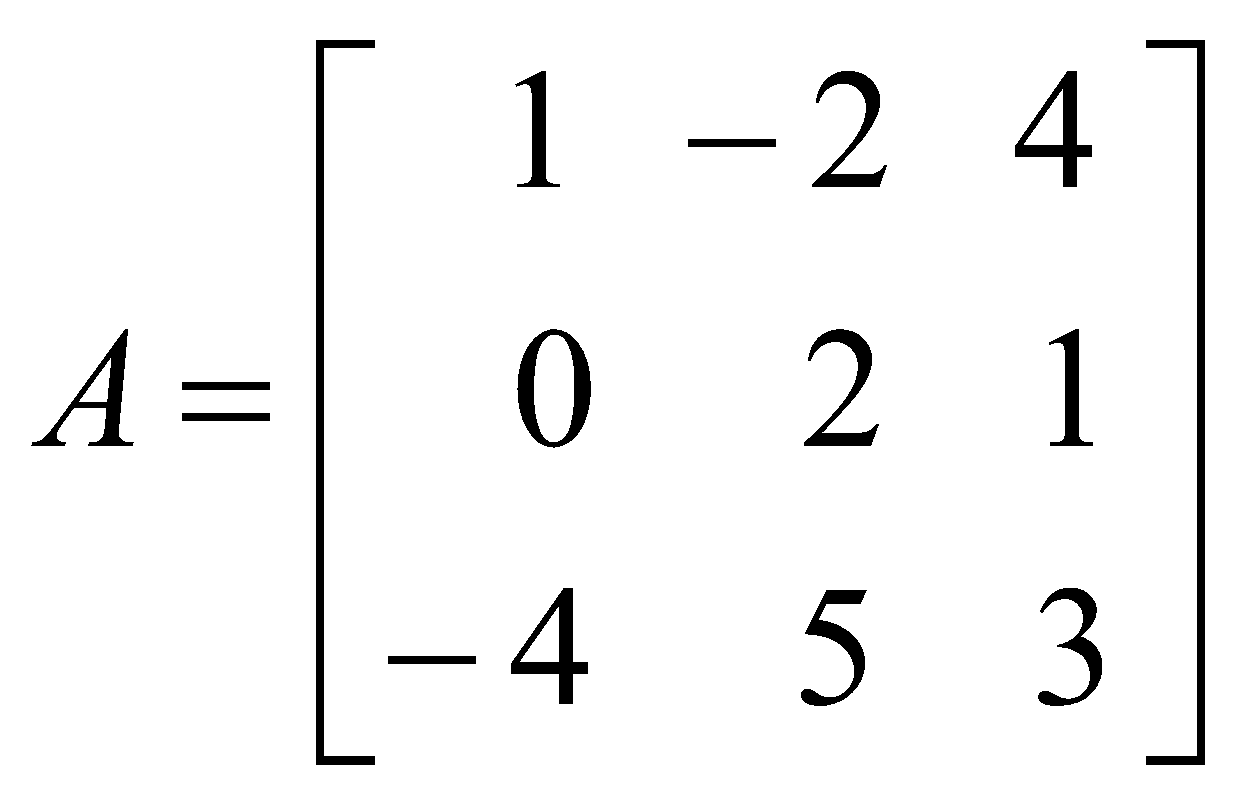(b) If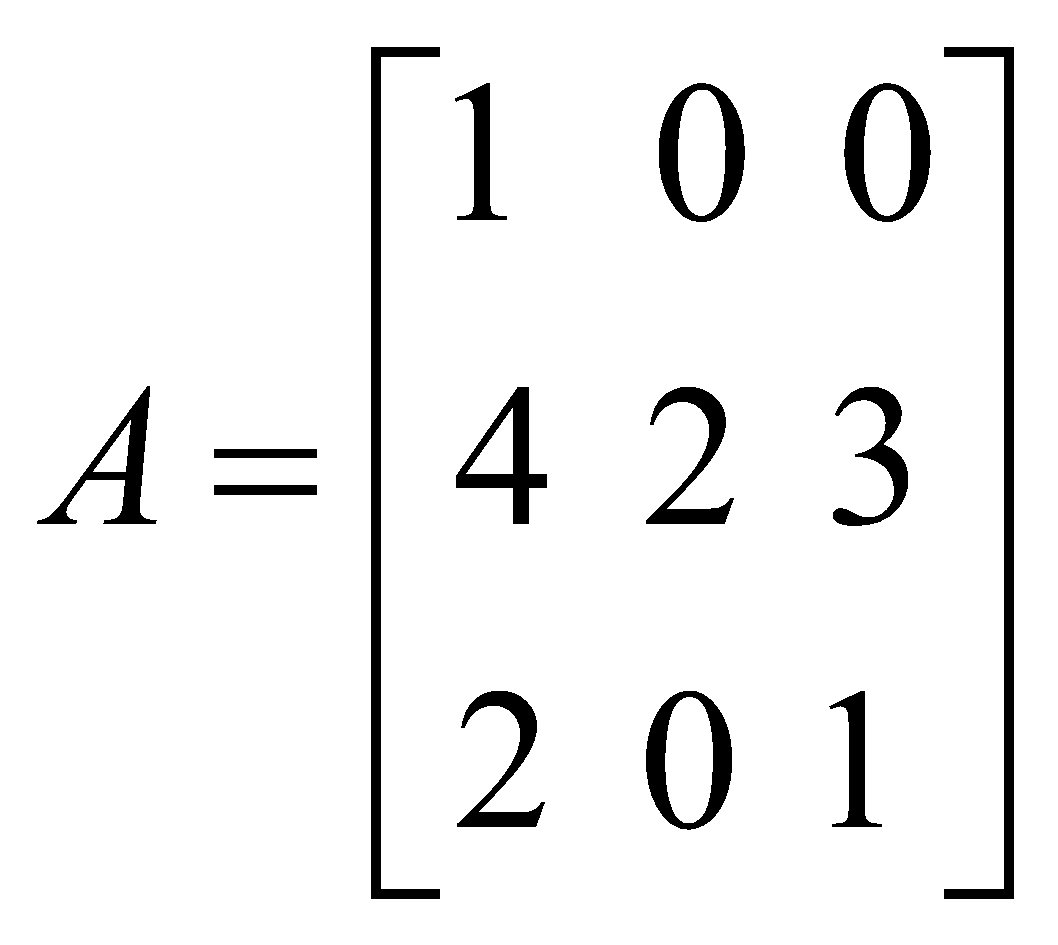4

Find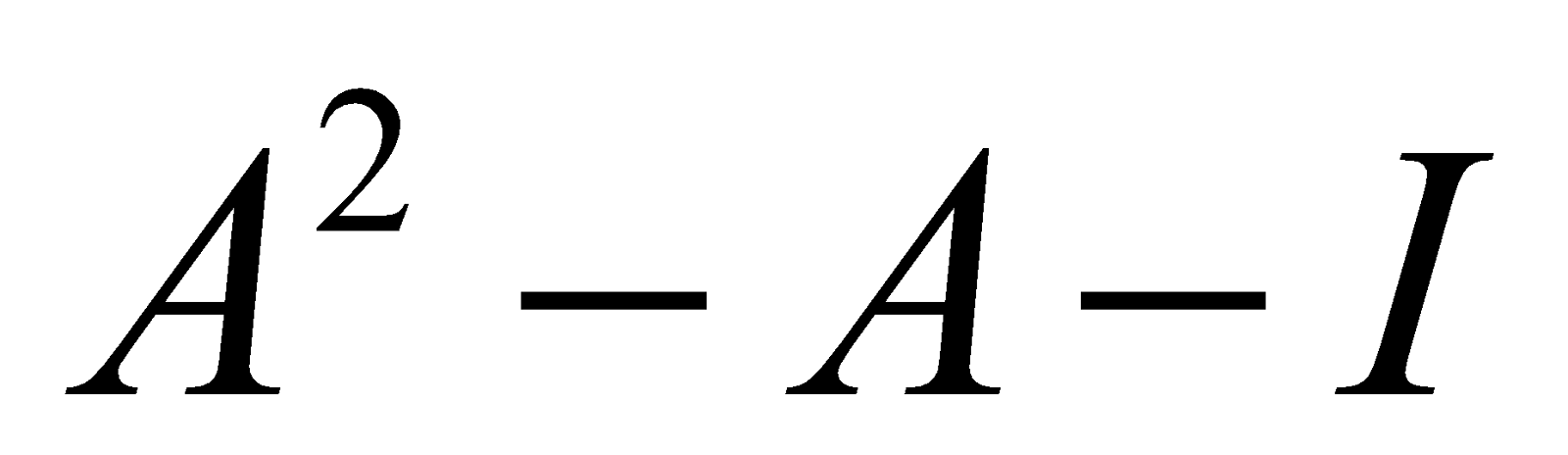.

Or

3. (a) Using matrices, solve the following equation: 4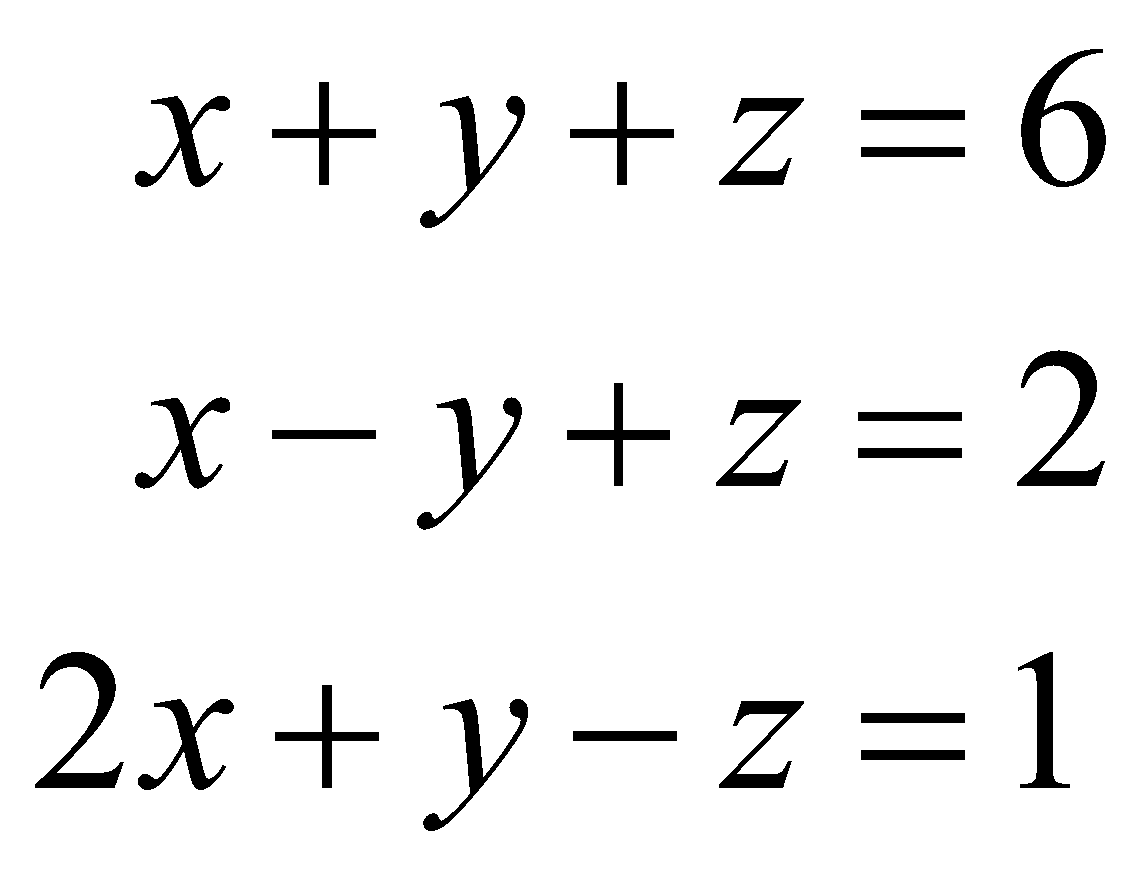(b) What is a square matrix?

(c) Define diagonal matrix and column matrix.

UNIT - II

4. Answer any two of the following questions: 2x2=4

1. State the distinction betweenand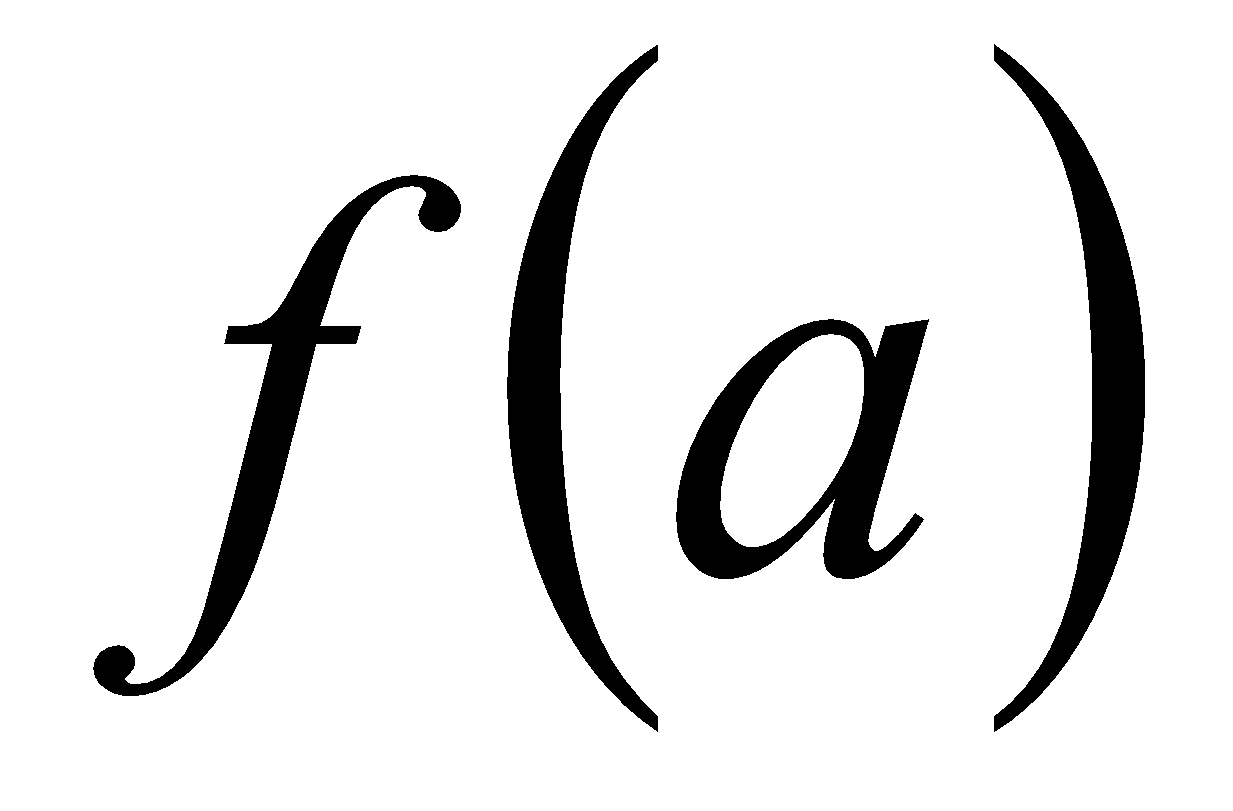2. Write the necessary and sufficient conditions for a function to be maximum.

3. If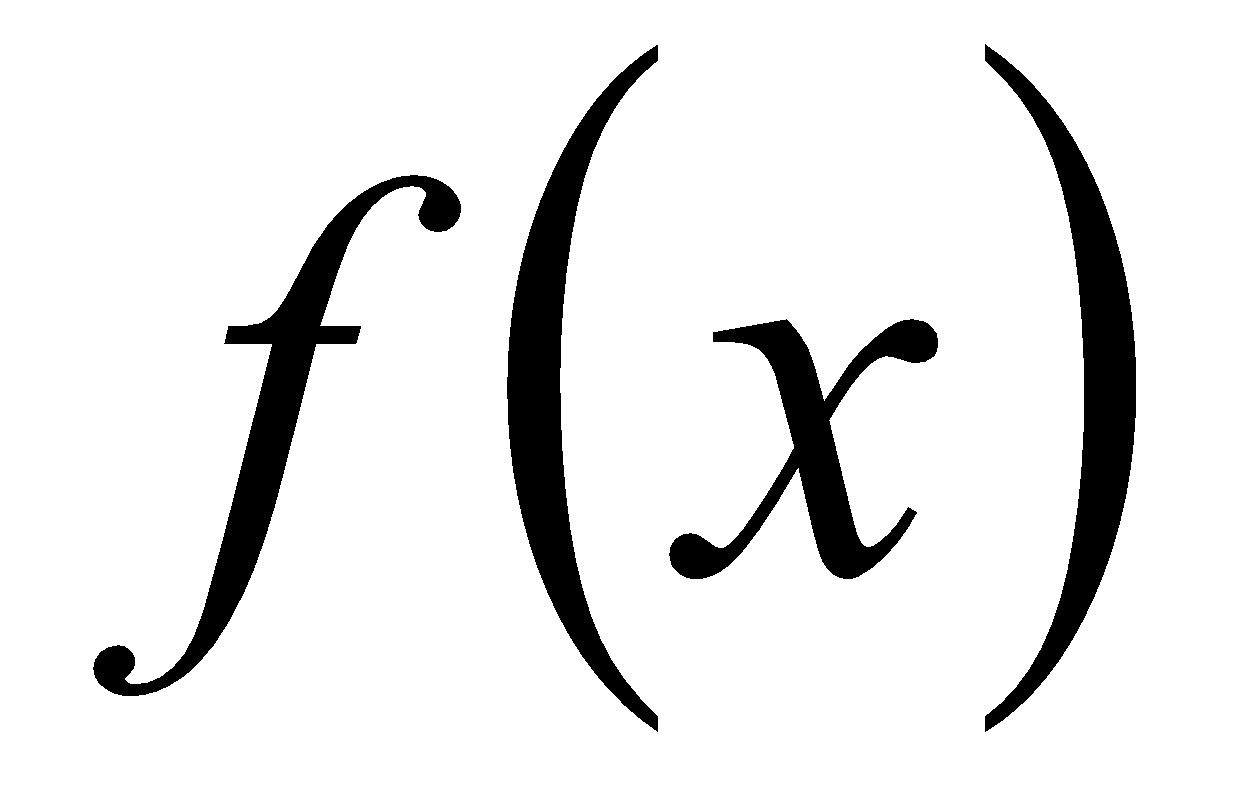and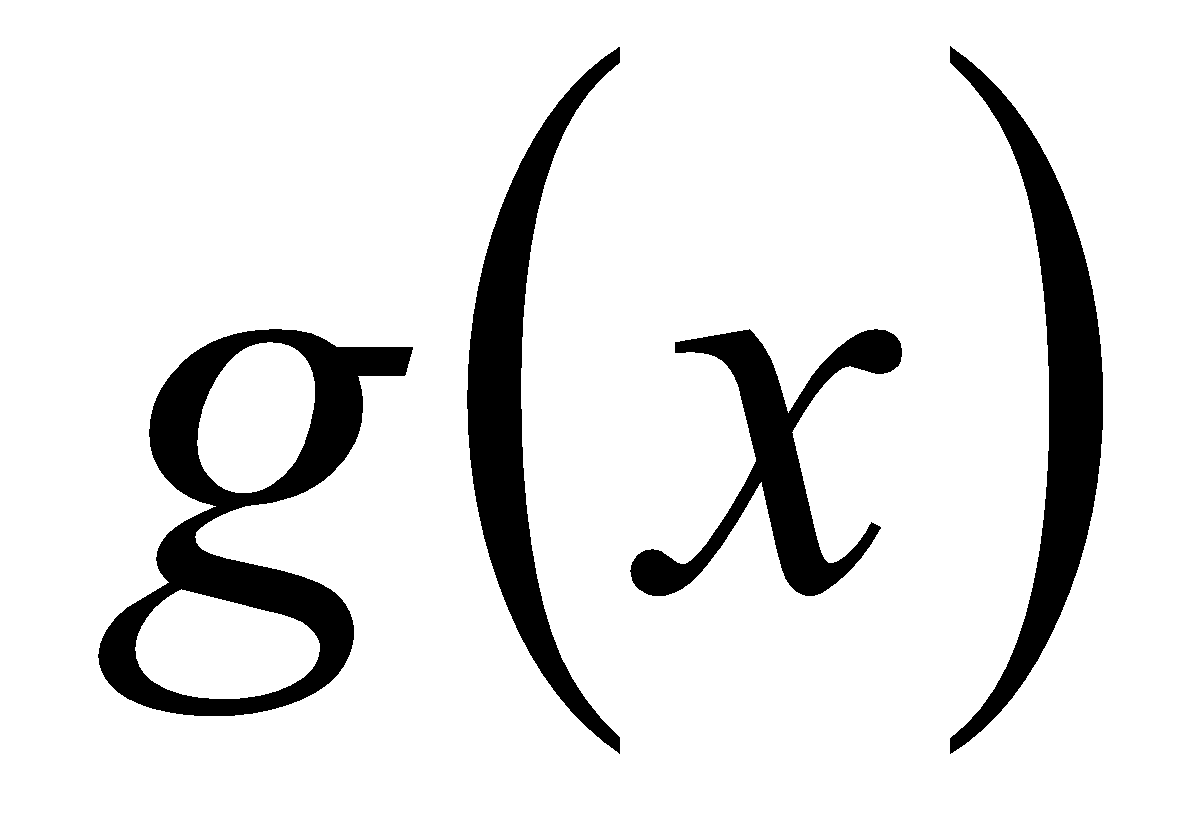are two functions of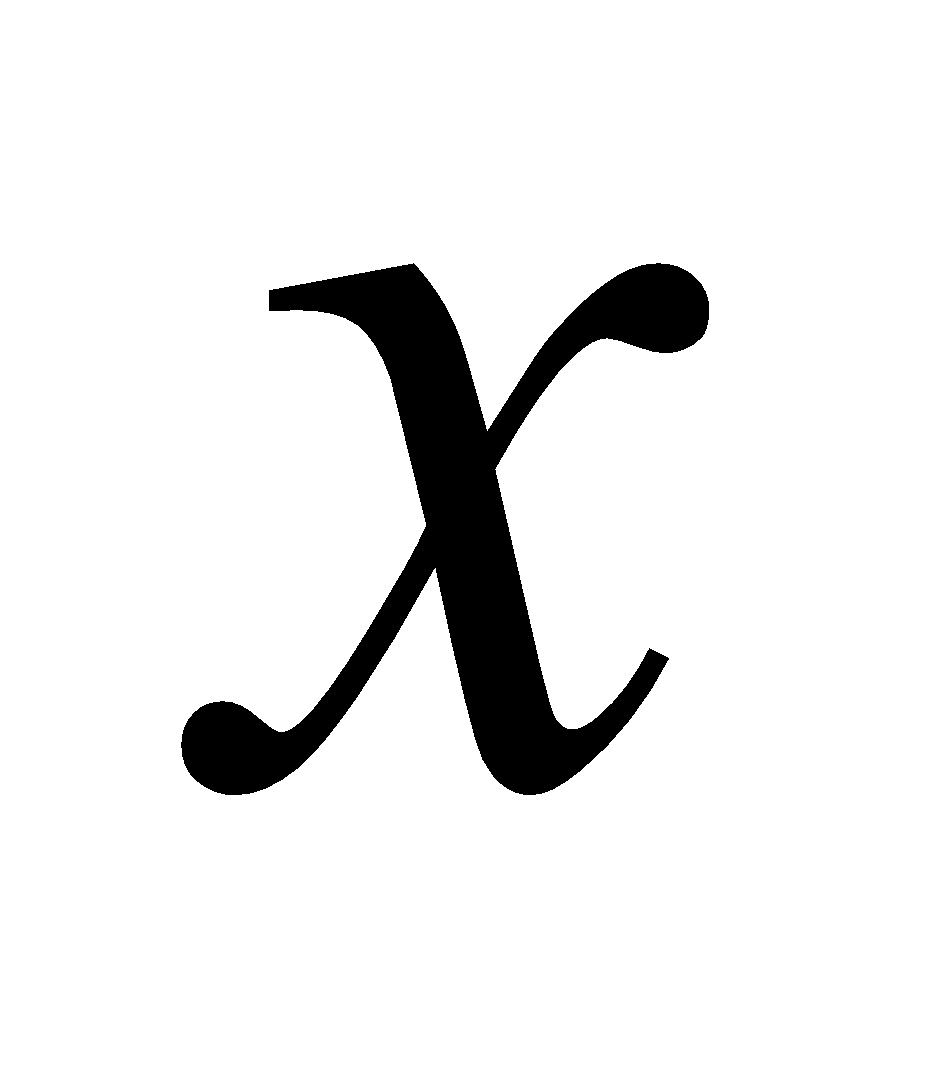, then find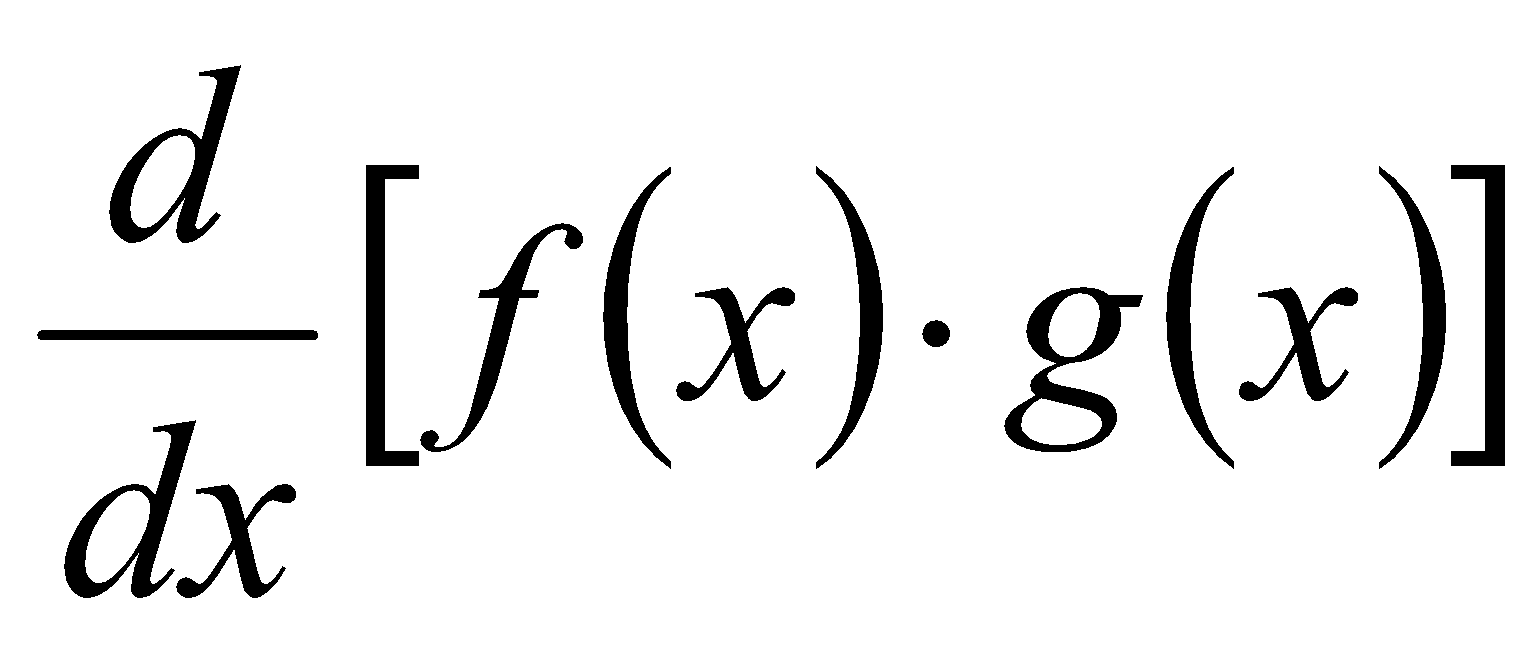5. (a) Discuss the continuity of the following function: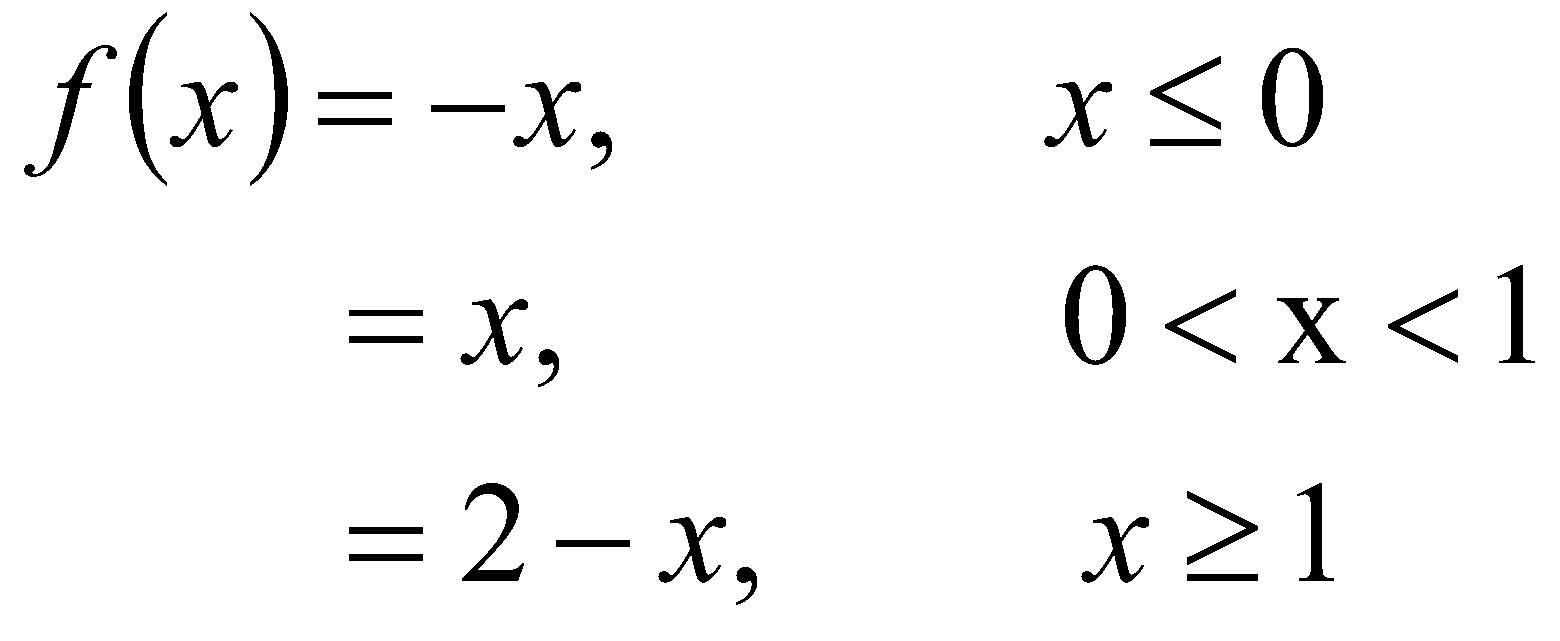At 0 and 1. 4

(b) Show that the function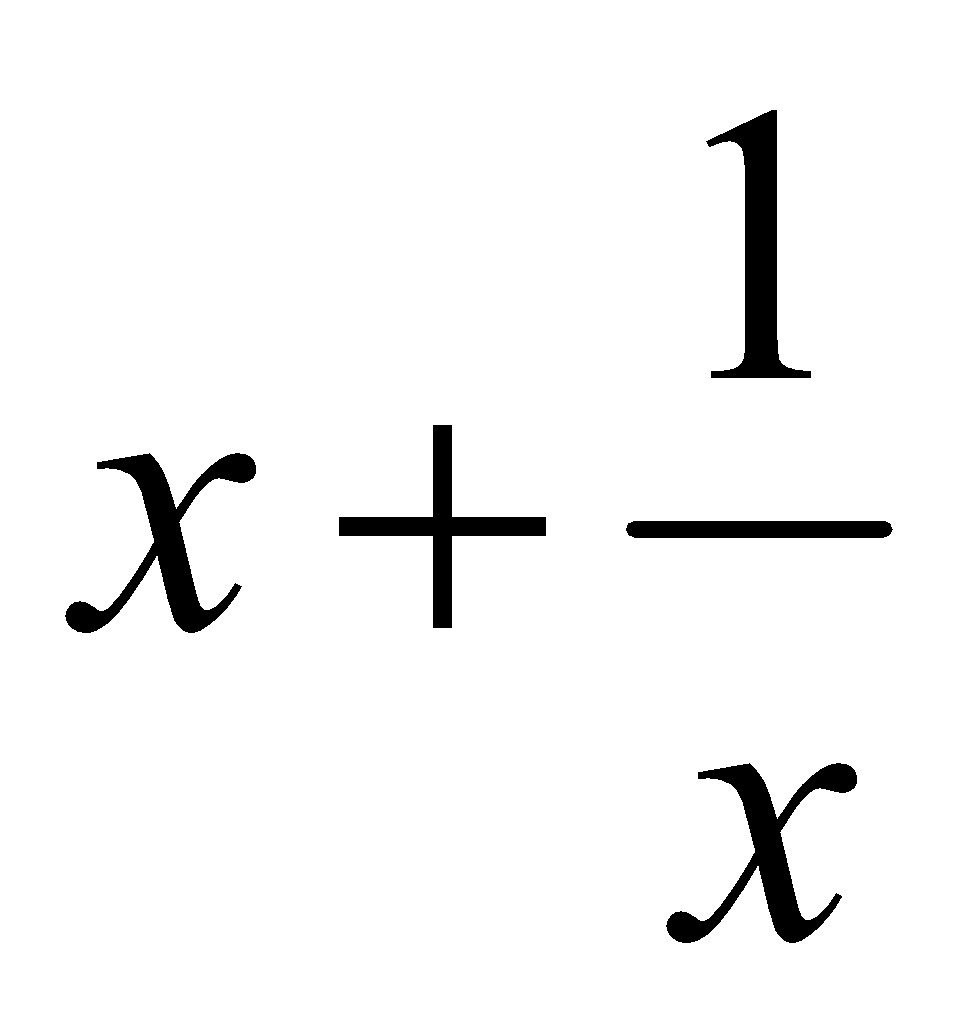has a maximum and minimum, but the maximum is less than the minimum.       4

(c) Evaluate: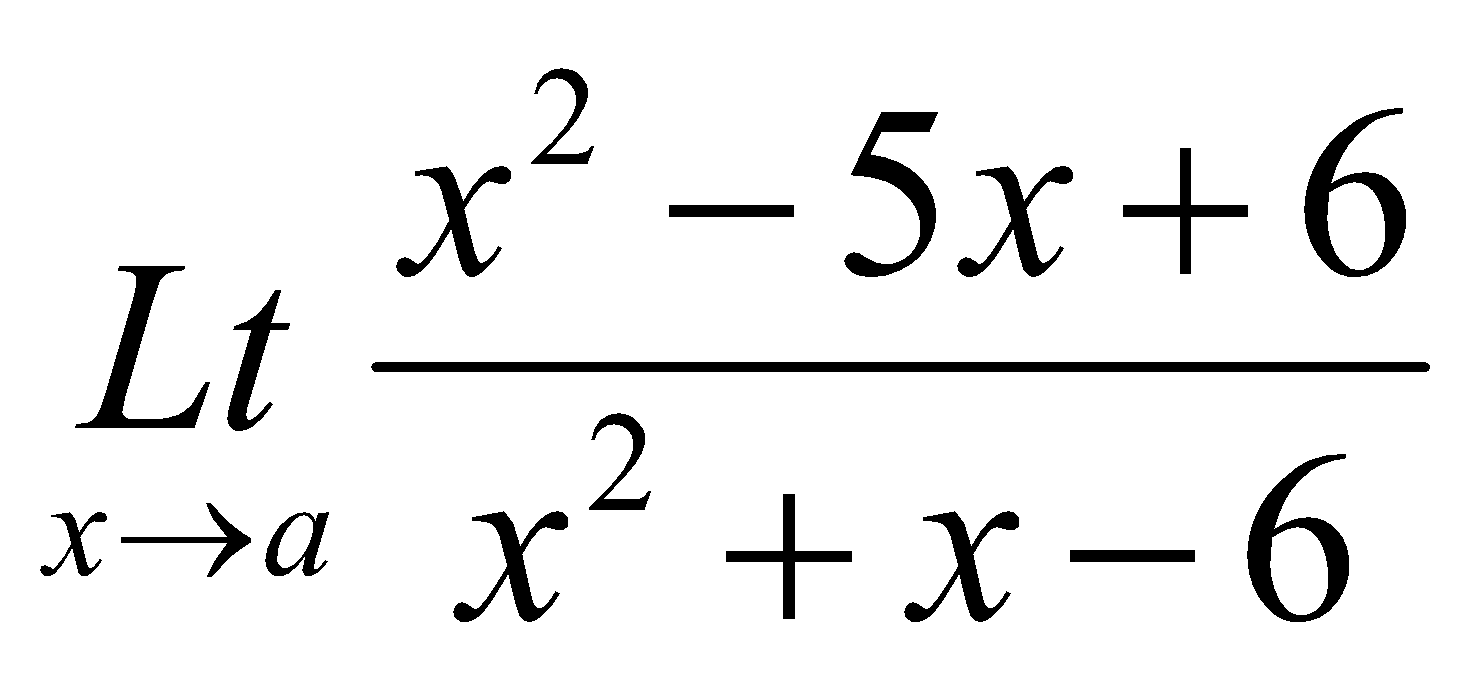2

Or

6. (a) Evaluate: 2x2=4

1.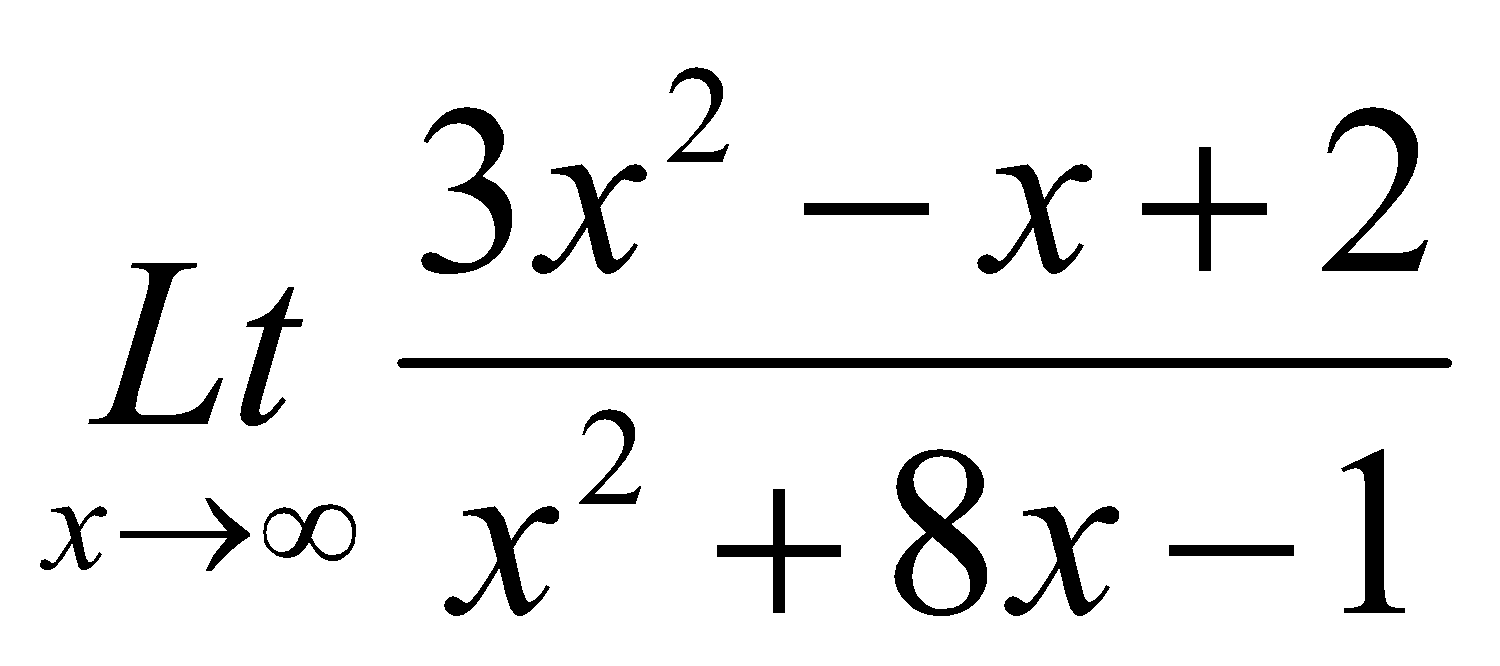2.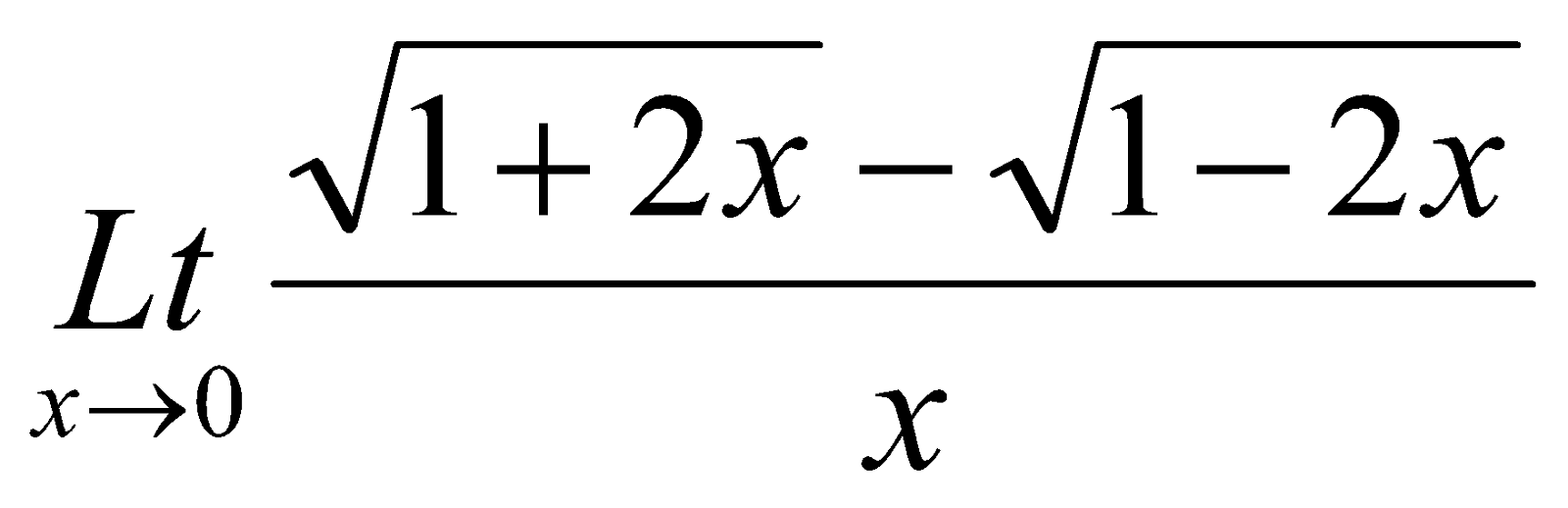(b) If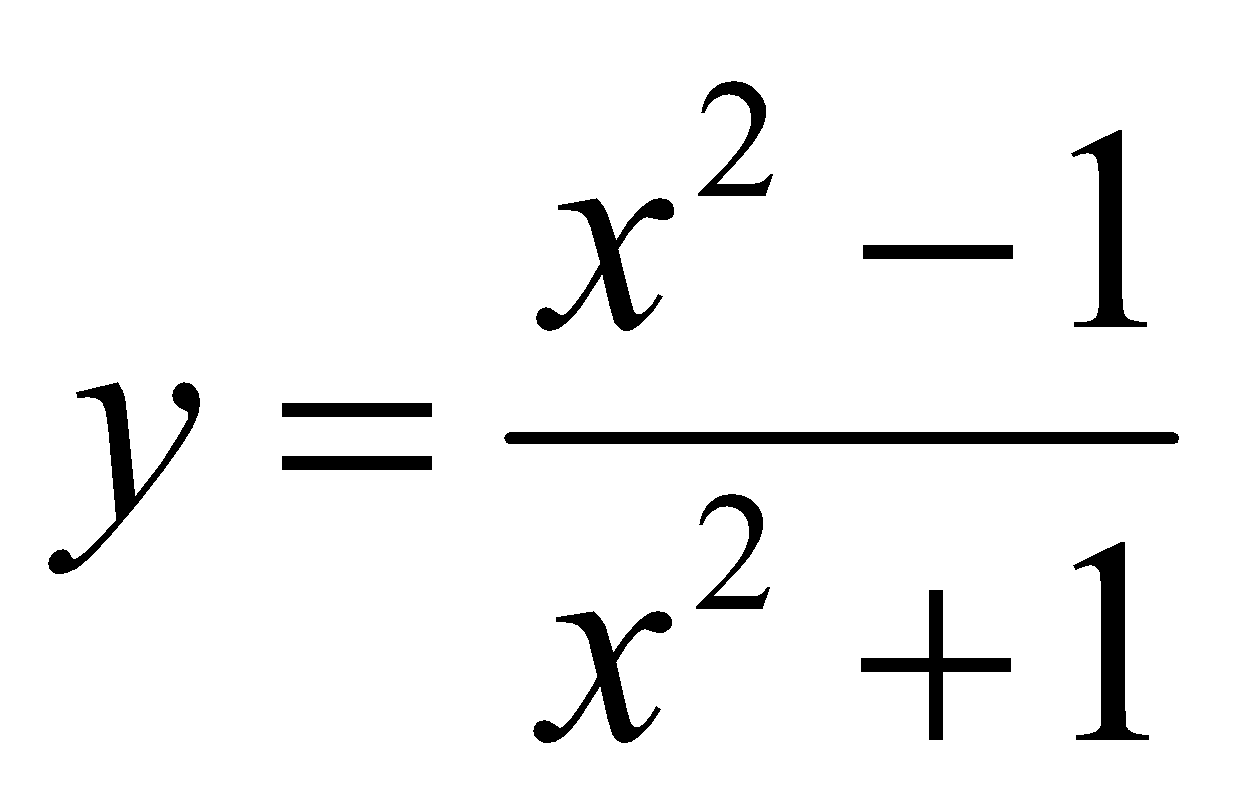, then find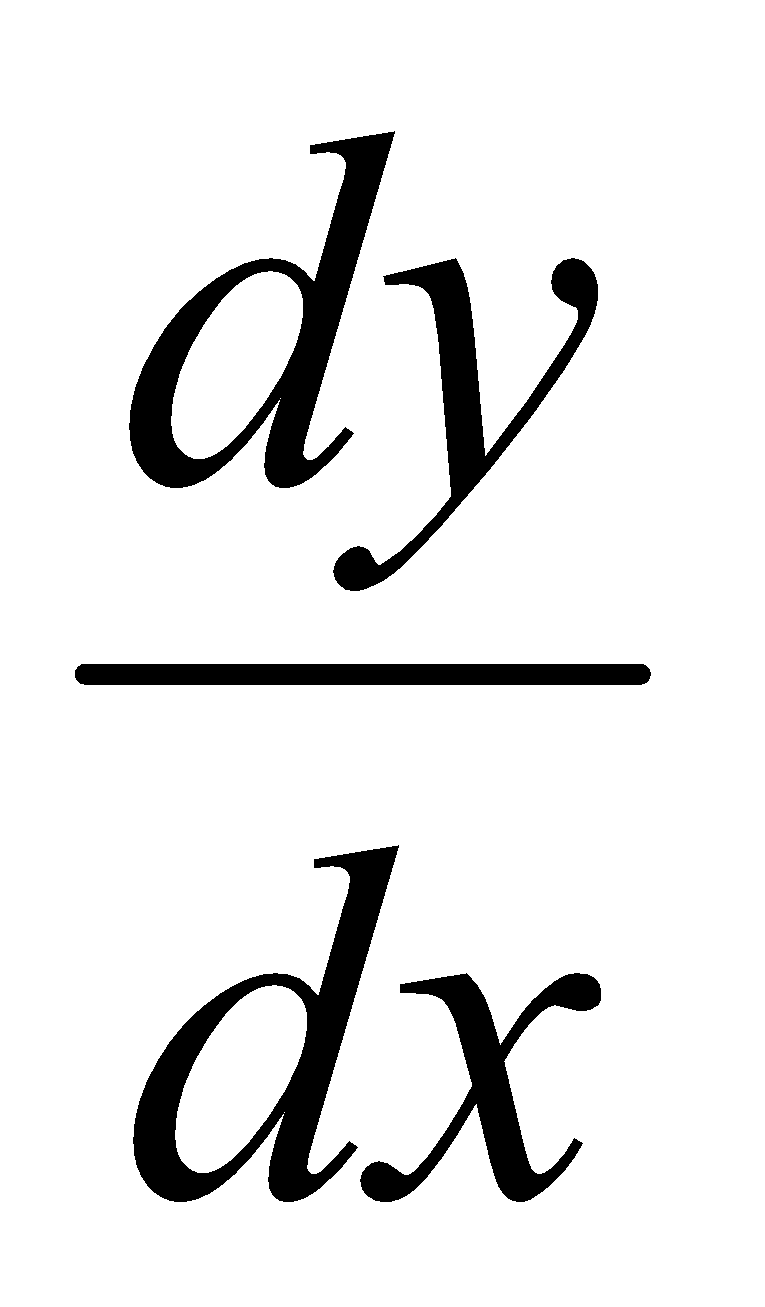. 3

(c) Find, if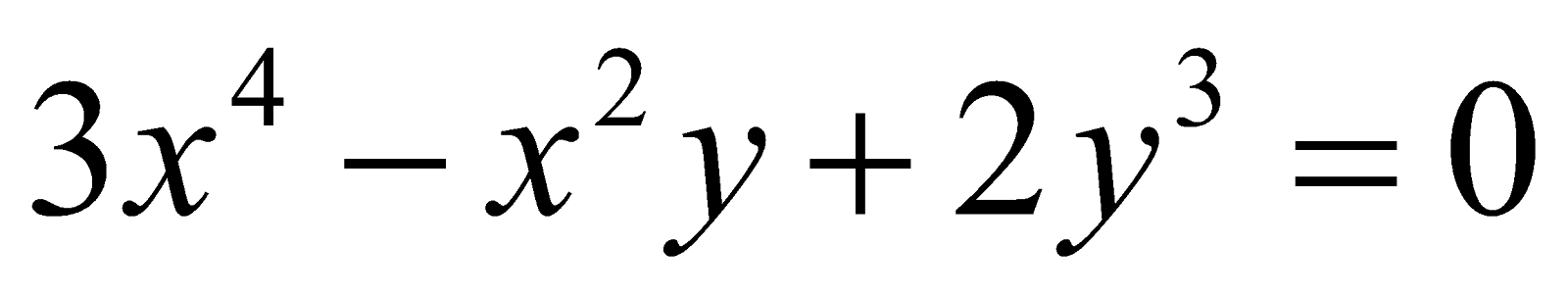. 3

UNIT – III

7. Answer any two of the following questions: 2x2=4

1. Write down the relation among mean, median and mode.

2. What is measure of variation?

3. Give any two examples of open-end classes.

8. Calculate mean, median, mode and standard deviation from the following data:

 Marks: 0 – 10 10 – 20 20 – 30 30 – 40 40 – 50 No. of Students: 3 5 10 17 20

 Marks: 50 – 60 60 – 70 70 – 80 80 – 90 90 – 100 No. of Students: 30 7 5 2 1

Or

9. From the data given in Q. No. 8, find the quartile deviation.

UNIT – IV

10. Answer any two of the following questions: 2x2=4

1. Interpret, r = 0 and r = +1.

2. Write down the regression equation of X on Y and Y on X.

3. Distinguish between correlation and regression (any two differences).

11. Calculate the correlation coefficient between the marks of Math and marks of Statistics:

 Marks of Math (X): 25 35 40 55 45 85 95 90 80 75 Marks of Statistics (Y): 35 45 30 45 55 75 85 70 90 85

Also find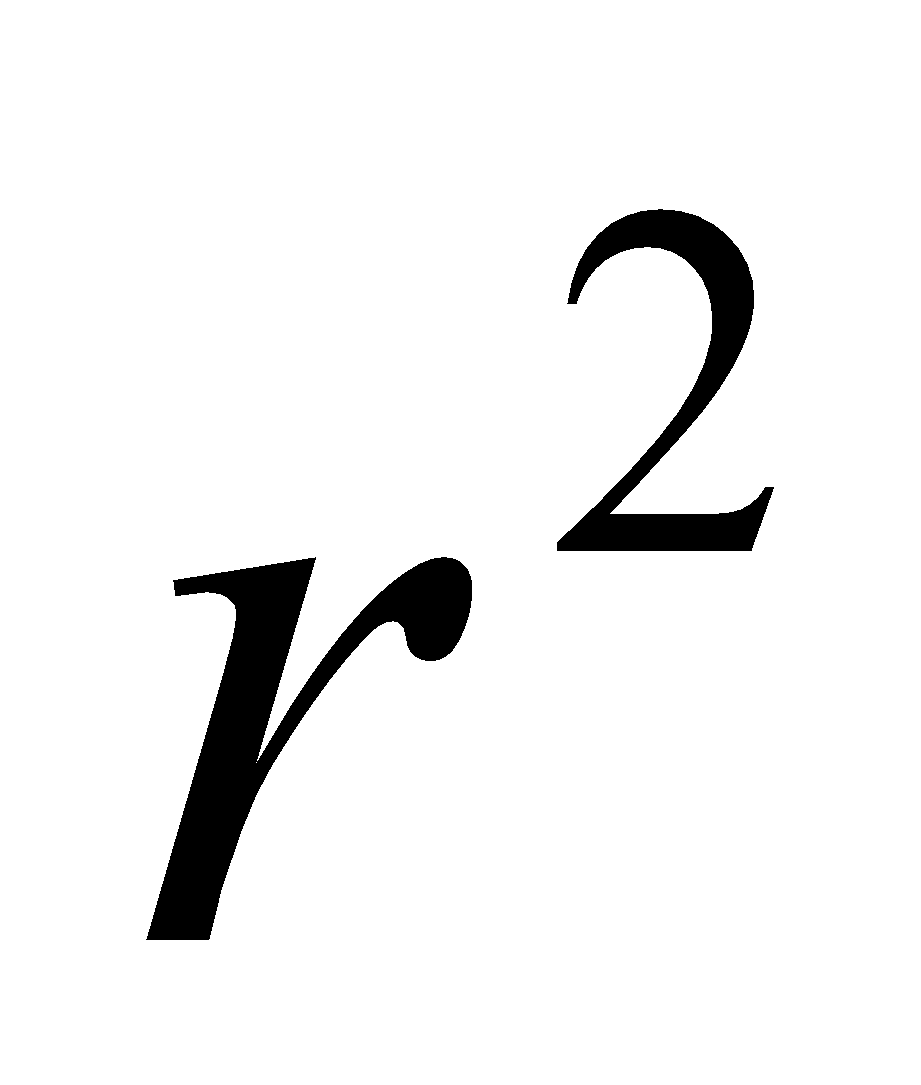. 8+2=10

Or

12. (a) Write a note on Spearman rank correlation. 5

(b) From the data given in Q. No. 11, calculate the regression coefficient of Y on X. 5

UNIT – V

13. Answer any two of the following questions: 2x2=4

1. Name two tests for ideal index number.

2. Give two examples of seasonal variation.

3. Give two examples of irregular variation.

14. (a) Fit a linear trend by the method of least squares:

 Year: 2000 2001 2002 2003 2004 2005 Production (in Rs. Crore): 7 10 12 14 17 24

(b) Write two drawbacks of the method of moving averages.

Or

15. (a) Write down the different steps for the construction of index number.

(b) Calculate Fisher’s ideal index number from the data given below:

 Base Year Current Year Commodities Price (in Rs.) Quantity(in kg) Price (in Rs.) Quantity (in Kg) ABCD 10203040 15202515 20302550 14182512

***